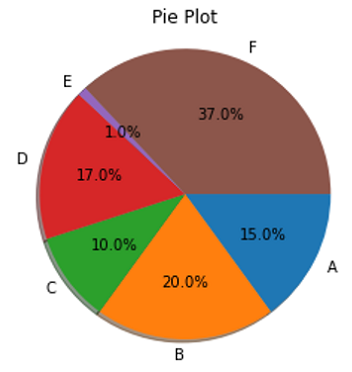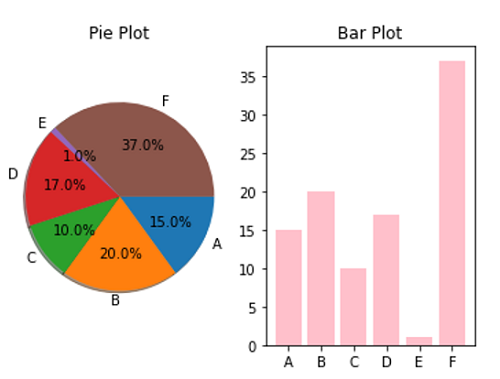# Python | Bar Plot vs Pie Plot

In this tutorial, we are going to compare Pie Plot and Bar Plot in terms of visualization.
Submitted by Anuj Singh, on August 04, 2020

We used Subplot for side by side plot comparison. This would help us to decide which plotting technique should be used for any desired application.

Pie: When the data to be summed up to 100 Percent then it is preferable to use Pie Plot

Bar: When we have to compare multiple categories but the sum is not 100, then it is good to use Bar Plot.

Illustration:## Python code to demonstrate example to compare bar plot and pie plot

```import matplotlib.pyplot as plt

labels = 'A', 'B', 'C', 'D', 'E', 'F'
sizes = [15, 20, 10, 17, 1, 37]
alp = (0.9, 0.9, 0.9, 0, 0.9, 0.9)
plt.figure()
plt.title('Pie Plot')
plt.axis('equal')
plt.show()

plt.figure()
plt.title('Bar Plot')
plt.bar(labels, sizes, color='pink')
plt.show()

plt.figure()
plt.subplot(121)
plt.title('Pie Plot')
plt.axis('equal')

plt.subplot(122)
plt.title('Bar Plot')
plt.bar(labels, sizes, color='pink')
plt.show()
```

Output:

```Output is as figure
```

Languages: » C » C++ » C++ STL » Java » Data Structure » C#.Net » Android » Kotlin » SQL
Web Technologies: » PHP » Python » JavaScript » CSS » Ajax » Node.js » Web programming/HTML
Solved programs: » C » C++ » DS » Java » C#
Aptitude que. & ans.: » C » C++ » Java » DBMS
Interview que. & ans.: » C » Embedded C » Java » SEO » HR
CS Subjects: » CS Basics » O.S. » Networks » DBMS » Embedded Systems » Cloud Computing
» Machine learning » CS Organizations » Linux » DOS
More: » Articles » Puzzles » News/Updates